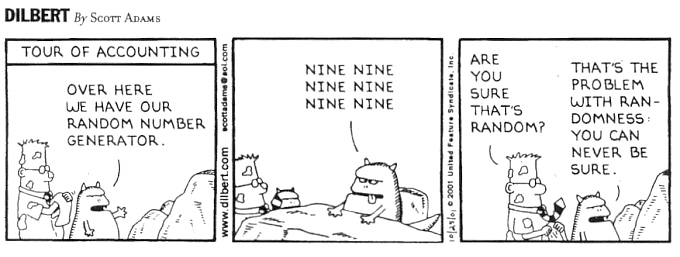# Applying Shannon Entropy to data storage

I know Shannon Entropy is defined for messages. When it's said the size of a file stored in a HDD is 1 MB, are we talking about the Shannon Entropy? If so, how do we extend the definition to static information (not flowing like messages)? If not, how come they have the same unit, i.e. bits?

• Do mean what Windows tells you? No, that's way more than the entropy. Which would be useless: you don't want to know how much information got stored, but how much space is occupied.
– Raphael
Apr 17, 2018 at 11:03
• If we compress the file to a Zip file, or maybe compress it as much as possible, do we get the entropy? Apr 17, 2018 at 11:13
• Still no. Every filesystem in practical use stores data with overhead. Read up on block size in this context. Also, zipping is not perfect; it doesn't even always increase entropy! Shannon entropy is a purely theoretical ideal.
– Raphael
Apr 17, 2018 at 12:04
• Now theoretically, is there an entropy associated with every digital file? Suppose we have an 10x10 image. If all the 100 pixels have the same RGB, it should have less entropy than if the pixels had random RGB. Can we calculate the entropy of such file? If so, how can we apply entropy to static files sitting on a HDD? Apr 17, 2018 at 15:48
• The Kolmogorov complexity of the file content when interpreted as a string may be closer to the measure you're looking for. Shannon entropy appears out of place here.
– Kai
Apr 18, 2018 at 10:07

No. That is telling you the size of the file, i.e., that's a statement about its length, not its entropy.

Of course, the length gives an upper bound on the entropy, but it is not itself a value for the entropy. The actual entropy could be smaller.

No, compressing the file does not tell you its entropy. The length after compression is, on average, a reasonable upper bound for the entropy -- but the true entropy could be much smaller.

Beware that it only makes sense to talk about the entropy of a random process or the entropy of a distribution (e.g., the entropy of a process used to generate a file), not the entropy of a single file. As an analogy, consider this old joke:It doesn't make sense to say that "the number nine is random"; that's a property of the process used to generate the number, not the number itself. You could get a 9 by rolling a ten-sided die (which is a random process), or you could get a 9 by writing the largest single-digit decimal number (that's not a random process). Similarly, it doesn't make sense to ask what is the entropy of the number nine; that is not well-defined. We can talk about the entropy of the random process that was used to generate it, though.